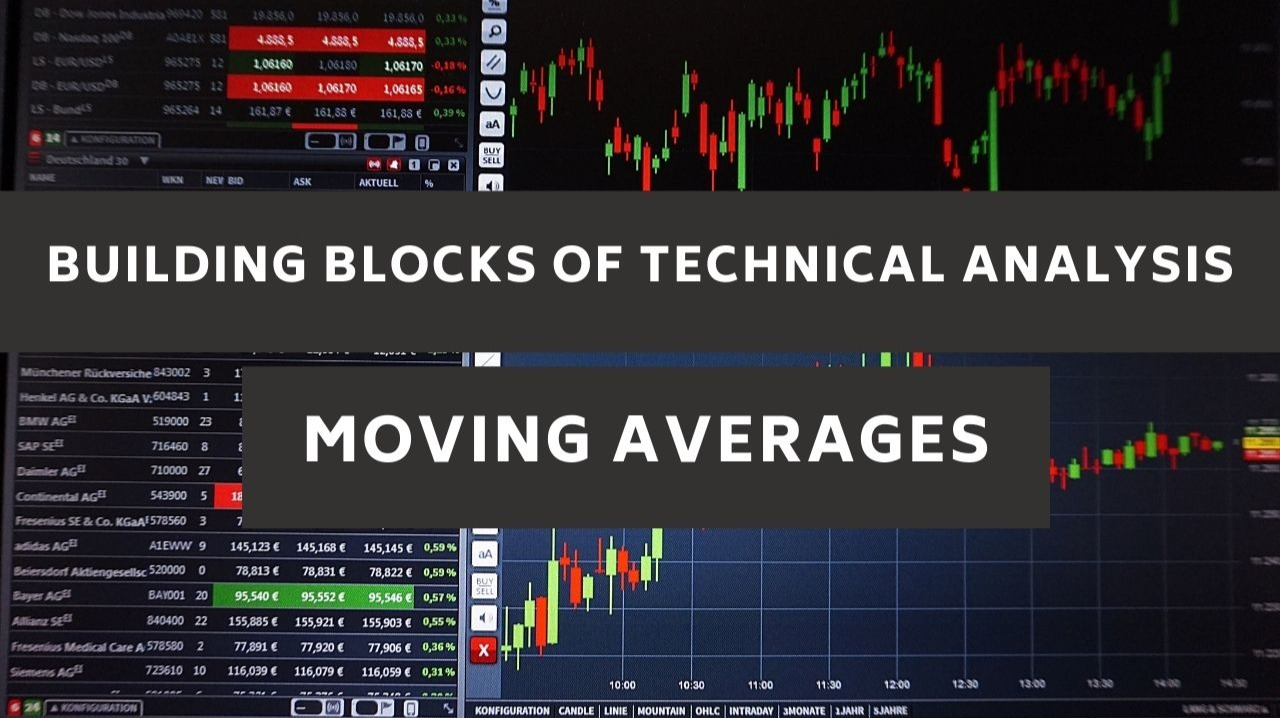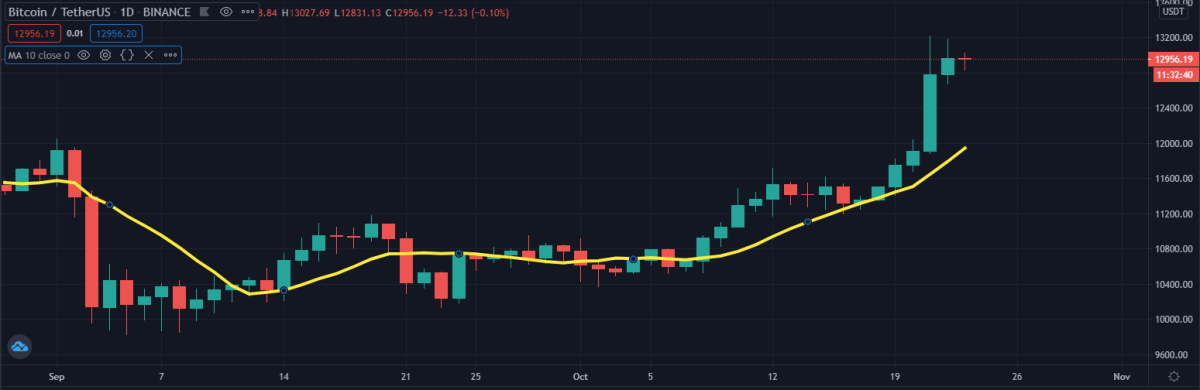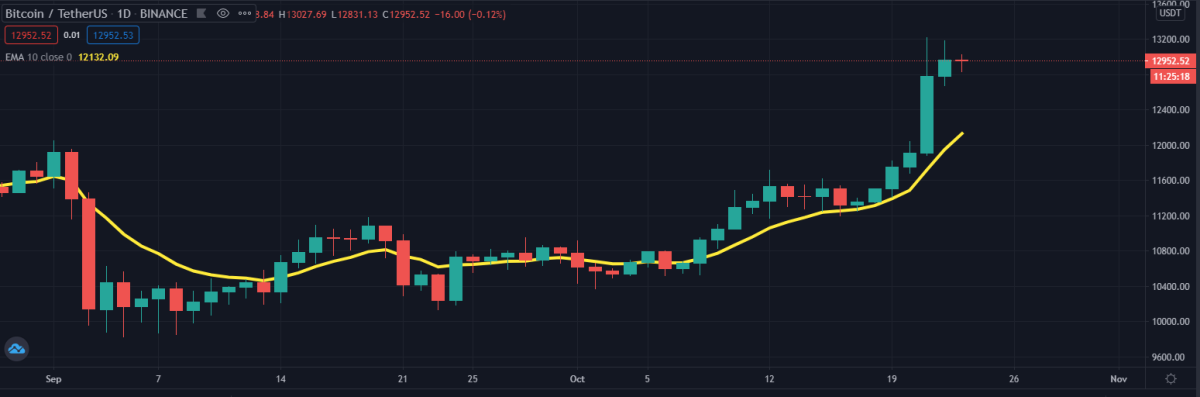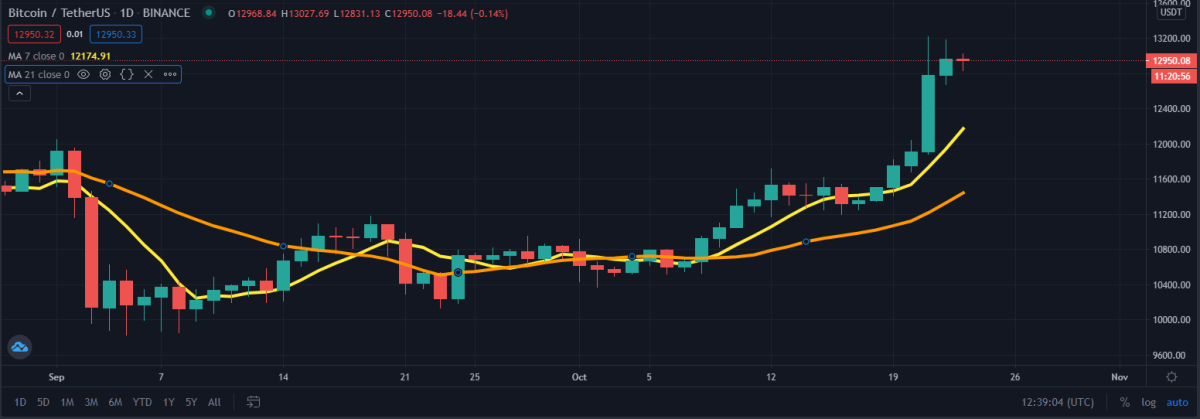Building Blocks of Technical Analysis - Moving Averages# Building Blocks of Technical Analysis - Moving Averages

By mercurial9 | mercurial9 | 23 Oct 2020

A multitude of different moving averages exist. The primary difference between the averages is the weight given to various data points, especially recent ones. The reasoning behind the weighting theory is that in assessing market movements, new data is increasingly more critical. The behavioral basis of technical analysis indicates that recent price changes should have a more significant impact on the psychology and decisions of market participants.

Definition: An indicator that shows the average value of a security or other data over a specified period. It is a smoothing technique that takes away noisy price action to indicate a probable price path.

## How to determine Moving Averages

The Simple Moving Average (SMA) and the Exponential Moving Average (EMA) are the two most common forms of moving averages. Below, they are described in more detail. To apply the technique effectively, it is, however, necessary to understand the basic calculations.

### Simple Moving Averages (SMA)

The simple moving average consists of a measure of the average (mean) security price over a given number of periods. Most moving averages are generated from the closing price. For example, by adding the closing prices for the last 5 days and dividing the total by 5, a 5-day simple moving average is calculated.

10 + 11 + 12 + 13 + 14 = 60
(60 / 5) = 12

To form a smooth curving line, i.e. the moving average line - the averages are then joined. Continuing with our example, if the average closing price is 15, then this new date will be added and the oldest day will be dropped, which is 10. The current simple moving average of 5 days will be calculated as follows.

11 + 12 + 13 + 14 +15 = 65
(65 / 5) = 13The price chart above illustrates the fact that lagging indicators are all moving averages and always "behind" the price. The market is on an uptrend, but the simple moving average, based on data from the previous 10 days, remains below the market. If the price were to increase, it would most probably be above the SMA. Since moving averages are lagging indicators, they match the trend following indicators. Moving averages work well when prices are trending. However, moving averages can offer misleading signals when prices are not trending.

### Exponential Moving Average (EMA)

Technical analysts also use exponential moving averages (also called exponentially weighted moving averages) to decrease the lag in simple moving averages. By adding more weight to recent prices compared to older prices, EMA's reduces the lag. The weighting applied to the most recent price depends on the moving average period defined. The shorter the time of the EMA, the greater the weight added to the most recent price.

#### Exponential Moving Average Calculation

There are two ways to calculate exponential moving averages - as a percent-based EMA or as a period-based EMA. As a single parameter, a percent-based EMA has a number, while a period-based EMA has a parameter that reflects the length of the EMA. In other words, an exponential moveable average adds a percentage of the closing price of today to a percentage of the EMA of yesterday.

The formula for an exponential moving average is: EMA (current) = ((Price(current) – EMA (prev)) x Multiplier) + EMA (prev)

Notice that, in principle, in the calculation of each EMA that makes up the EMA line, every previous closing price in the data set is used. Although the influence of older data points diminishes with time, it never disappears entirely. Regardless of the EMA's stated time, this is true. For shorter EMAs than for longer ones, the effects of older data decrease quickly, but, again, they never entirely vanish.### How to apply and interpret moving averages

Essentially, the moving average is a trend following strategy. The primary objective is to acknowledge a new trend or that an existing trend is shifting or reversing. Plotting a single moving average on the price chart is the most widely used strategy. When the price passes the moving average, a possible trend in the direction of the most recent price change is suggested. Therefore, if the price rises above the moving average or a 'sale' signal if the price crosses below the moving average, this may be a 'purchase' signal. It remains part of the technical analyst competence to choose how long and what sort of moving average is used.

A second common method is called the double crossover method, where two moving averages, one short-term and one long-term, are constructed. More prone to shifts in the underlying price is the shorter-term average. This implies that the pattern would follow the short-term moving average when the short-term moving average exceeds the long-term moving average. With the significant event being the crossing of the averages, the short-term moving average thus becomes a smooth proxy for the price data.### Strengths and disadvantages

• As a smooth data sequence, moving averages are a relatively easy method that makes it easier to recognize patterns.
• The levels describing the pattern are quantifiable. In automated trading systems and algorithmic trading, defining a crossover event is useful. This also tackles the subjective flaw of trend line analysis that is inherent.
• Construction blocks for many other technical indicators and overlays are used.
• Moving averages are known to be trend measures that match or lag, and any signal would be delayed. Moving averages can not forecast a pattern shift but follow the present trend instead. Therefore, not for prediction, they are better suited for trend detection and trend tracking purposes.
• While moving averages work well in trending markets, in sideways and ranging environments, they appear to send false signals.

## Concluding thoughts

What moving average you use will depend on your style and preferences for trading and investing. There is undoubtedly a lag in the simple moving average, but the exponential moving average might be more susceptible to 'whiplashing.' To capture changes faster, some traders tend to use exponential moving averages for shorter periods. To detect long-term pattern shifts, some investors prefer simple moving averages over long periods. In an active trading portfolio, a 50-day SMA might work well to define support levels, but a 100-day EMA may work better for a more passive approach. The moving average form and length of time would depend significantly on the protection of the person and how it has responded in the past.

For others, the initial thought is that greater sensitivity and faster signals are bound to be advantageous. For the technical analyst, this is not always accurate and brings up a great dilemma: the trade-off between sensitivity and reliability. The more sensitive an indicator is, the more signals will be given. These signals may prove timely, but an increase in false signals comes with increased sensitivity. The less sensitive an indicator is, the fewer signals will be given. Less sensitivity does, however, contribute to less and more accurate signals. These signals may also be late occasionally.

The same problem relates to moving averages. It will be more susceptible to shorter moving averages and produce more signals. More signals will also likely be produced by the EMA, which is usually more sensitive than the SMA. However, the number of false signals and whipsaws will also increase. Longer moving averages are going to travel slower and show fewer signals. These signals may prove more accurate, but they may also arrive late. To explore the trade-off between sensitivity and signal reliability, every investor or trader should experiment with distinct moving average lengths and forms.

Thank you for reading and hope you have a good rest of the day!

I also post my content on the following platforms:
Publish0x: https://www.publish0x.com/@aftershock9?a=Vyb82ANmev
Hive: https://hive.blog/@mercurial9
Read.cash: https://read.cash/@merurial9
Uptrennd: https://www.uptrennd.com/user/mercurial9
Steempeak: https://steempeak.com/@mercurial9
Instagram: https://www.instagram.com/minimalistpixel/
Pinterest: https://za.pinterest.com/minimalistpixel/boards/
Twitter: https://twitter.com/minimalistpixelmercurial9

Learning about crypto and crypto gaming.mercurial9

Learning about crypto, crypto gaming and techSend a \$0.01 microtip in crypto to the author, and earn yourself as you read!

20% to author / 80% to me.
We pay the tips from our rewards pool.